# PySpark Groupby

• Last Updated : 19 Dec, 2021

In this article, we are going to discuss Groupby function in PySpark using Python.

## Python3

 `# importing module``import` `pyspark`` ` `# importing sparksession from pyspark.sql module``from` `pyspark.sql ``import` `SparkSession`` ` `# creating sparksession and giving an app name``spark ``=` `SparkSession.builder.appName(``'sparkdf'``).getOrCreate()`` ` `# list  of student  data``data ``=` `[[``"1"``, ``"sravan"``, ``"IT"``, ``45000``],``        ``[``"2"``, ``"ojaswi"``, ``"CS"``, ``85000``],``        ``[``"3"``, ``"rohith"``, ``"CS"``, ``41000``],``        ``[``"4"``, ``"sridevi"``, ``"IT"``, ``56000``],``        ``[``"5"``, ``"bobby"``, ``"ECE"``, ``45000``],``        ``[``"6"``, ``"gayatri"``, ``"ECE"``, ``49000``],``        ``[``"7"``, ``"gnanesh"``, ``"CS"``, ``45000``],``        ``[``"8"``, ``"bhanu"``, ``"Mech"``, ``21000``]``        ``]`` ` `# specify column names``columns ``=` `[``'ID'``, ``'NAME'``, ``'DEPT'``, ``'FEE'``]`` ` `# creating a dataframe from the lists of data``dataframe ``=` `spark.createDataFrame(data, columns)`` ` `# display``dataframe.show()`

Output: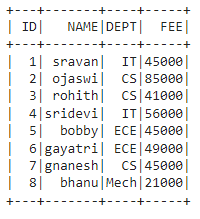In PySpark,  groupBy() is used to collect the identical data into groups on the PySpark DataFrame and perform aggregate functions on the grouped data

### The aggregation operation includes:

• count(): This will return the count of rows for each group.

dataframe.groupBy(‘column_name_group’).count()

• mean(): This will return the mean of values for each group.

dataframe.groupBy(‘column_name_group’).mean(‘column_name’)

• max(): This will return the maximum of values for each group.

dataframe.groupBy(‘column_name_group’).max(‘column_name’)

• min(): This will return the minimum of values for each group.

dataframe.groupBy(‘column_name_group’).min(‘column_name’)

• sum(): This will return the total values for each group.

dataframe.groupBy(‘column_name_group’).sum(‘column_name’)

• avg(): This will return the average for values for each group.

dataframe.groupBy(‘column_name_group’).avg(‘column_name’).show()

We have to use any one of the functions with groupby while using the method

Syntax: dataframe.groupBy(‘column_name_group’).aggregate_operation(‘column_name’)

### Example 1: Groupby with sum()

Groupby with DEPT along FEE with sum().

## Python3

 `# importing module``import` `pyspark`` ` `# importing sparksession from pyspark.sql module``from` `pyspark.sql ``import` `SparkSession`` ` `# creating sparksession and giving an app name``spark ``=` `SparkSession.builder.appName(``'sparkdf'``).getOrCreate()`` ` `# list  of student  data``data ``=` `[[``"1"``, ``"sravan"``, ``"IT"``, ``45000``],``        ``[``"2"``, ``"ojaswi"``, ``"CS"``, ``85000``],``        ``[``"3"``, ``"rohith"``, ``"CS"``, ``41000``],``        ``[``"4"``, ``"sridevi"``, ``"IT"``, ``56000``],``        ``[``"5"``, ``"bobby"``, ``"ECE"``, ``45000``],``        ``[``"6"``, ``"gayatri"``, ``"ECE"``, ``49000``],``        ``[``"7"``, ``"gnanesh"``, ``"CS"``, ``45000``],``        ``[``"8"``, ``"bhanu"``, ``"Mech"``, ``21000``]``        ``]`` ` `# specify column names``columns ``=` `[``'ID'``, ``'NAME'``, ``'DEPT'``, ``'FEE'``]`` ` `# creating a dataframe from the lists of data``dataframe ``=` `spark.createDataFrame(data, columns)`` ` `# Groupby with DEPT along FEE with sum()``dataframe.groupBy(``'DEPT'``).``sum``(``'FEE'``).show()`

Output: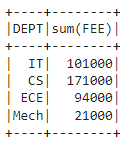## Python3

 `# importing module``import` `pyspark`` ` `# importing sparksession from pyspark.sql module``from` `pyspark.sql ``import` `SparkSession`` ` `# creating sparksession and giving an app name``spark ``=` `SparkSession.builder.appName(``'sparkdf'``).getOrCreate()`` ` `# list  of student  data``data ``=` `[[``"1"``, ``"sravan"``, ``"IT"``, ``45000``],``        ``[``"2"``, ``"ojaswi"``, ``"CS"``, ``85000``],``        ``[``"3"``, ``"rohith"``, ``"CS"``, ``41000``],``        ``[``"4"``, ``"sridevi"``, ``"IT"``, ``56000``],``        ``[``"5"``, ``"bobby"``, ``"ECE"``, ``45000``],``        ``[``"6"``, ``"gayatri"``, ``"ECE"``, ``49000``],``        ``[``"7"``, ``"gnanesh"``, ``"CS"``, ``45000``],``        ``[``"8"``, ``"bhanu"``, ``"Mech"``, ``21000``]``        ``]`` ` `# specify column names``columns ``=` `[``'ID'``, ``'NAME'``, ``'DEPT'``, ``'FEE'``]`` ` `# creating a dataframe from the lists of data``dataframe ``=` `spark.createDataFrame(data, columns)`` ` `# Groupby with DEPT along FEE with min()``dataframe.groupBy(``'DEPT'``).``min``(``'FEE'``).show()`

Output: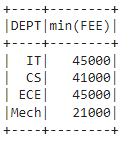## Python3

 `# importing module``import` `pyspark`` ` `# importing sparksession from pyspark.sql module``from` `pyspark.sql ``import` `SparkSession`` ` `# creating sparksession and giving an app name``spark ``=` `SparkSession.builder.appName(``'sparkdf'``).getOrCreate()`` ` `# list  of student  data``data ``=` `[[``"1"``, ``"sravan"``, ``"IT"``, ``45000``],``        ``[``"2"``, ``"ojaswi"``, ``"CS"``, ``85000``],``        ``[``"3"``, ``"rohith"``, ``"CS"``, ``41000``],``        ``[``"4"``, ``"sridevi"``, ``"IT"``, ``56000``],``        ``[``"5"``, ``"bobby"``, ``"ECE"``, ``45000``],``        ``[``"6"``, ``"gayatri"``, ``"ECE"``, ``49000``],``        ``[``"7"``, ``"gnanesh"``, ``"CS"``, ``45000``],``        ``[``"8"``, ``"bhanu"``, ``"Mech"``, ``21000``]``        ``]`` ` `# specify column names``columns ``=` `[``'ID'``, ``'NAME'``, ``'DEPT'``, ``'FEE'``]`` ` `# creating a dataframe from the lists of data``dataframe ``=` `spark.createDataFrame(data, columns)`` ` `# Groupby with DEPT along FEE with max()``dataframe.groupBy(``'DEPT'``).``max``(``'FEE'``).show()`

Output: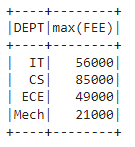## Python3

 `# importing module``import` `pyspark`` ` `# importing sparksession from pyspark.sql module``from` `pyspark.sql ``import` `SparkSession`` ` `# creating sparksession and giving an app name``spark ``=` `SparkSession.builder.appName(``'sparkdf'``).getOrCreate()`` ` `# list  of student  data``data ``=` `[[``"1"``, ``"sravan"``, ``"IT"``, ``45000``],``        ``[``"2"``, ``"ojaswi"``, ``"CS"``, ``85000``],``        ``[``"3"``, ``"rohith"``, ``"CS"``, ``41000``],``        ``[``"4"``, ``"sridevi"``, ``"IT"``, ``56000``],``        ``[``"5"``, ``"bobby"``, ``"ECE"``, ``45000``],``        ``[``"6"``, ``"gayatri"``, ``"ECE"``, ``49000``],``        ``[``"7"``, ``"gnanesh"``, ``"CS"``, ``45000``],``        ``[``"8"``, ``"bhanu"``, ``"Mech"``, ``21000``]``        ``]`` ` `# specify column names``columns ``=` `[``'ID'``, ``'NAME'``, ``'DEPT'``, ``'FEE'``]`` ` `# creating a dataframe from the lists of data``dataframe ``=` `spark.createDataFrame(data, columns)`` ` `# Groupby with DEPT along FEE with avg()``dataframe.groupBy(``'DEPT'``).avg(``'FEE'``).show()`

Output: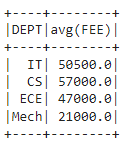## Python3

 `# importing module``import` `pyspark`` ` `# importing sparksession from pyspark.sql module``from` `pyspark.sql ``import` `SparkSession`` ` `# creating sparksession and giving an app name``spark ``=` `SparkSession.builder.appName(``'sparkdf'``).getOrCreate()`` ` `# list  of student  data``data ``=` `[[``"1"``, ``"sravan"``, ``"IT"``, ``45000``],``        ``[``"2"``, ``"ojaswi"``, ``"CS"``, ``85000``],``        ``[``"3"``, ``"rohith"``, ``"CS"``, ``41000``],``        ``[``"4"``, ``"sridevi"``, ``"IT"``, ``56000``],``        ``[``"5"``, ``"bobby"``, ``"ECE"``, ``45000``],``        ``[``"6"``, ``"gayatri"``, ``"ECE"``, ``49000``],``        ``[``"7"``, ``"gnanesh"``, ``"CS"``, ``45000``],``        ``[``"8"``, ``"bhanu"``, ``"Mech"``, ``21000``]``        ``]`` ` `# specify column names``columns ``=` `[``'ID'``, ``'NAME'``, ``'DEPT'``, ``'FEE'``]`` ` `# creating a dataframe from the lists of data``dataframe ``=` `spark.createDataFrame(data, columns)`` ` `# Groupby with DEPT  with count()``dataframe.groupBy(``'DEPT'``).count().show()`

Output: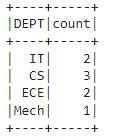## Python3

 `# importing module``import` `pyspark`` ` `# importing sparksession from pyspark.sql module``from` `pyspark.sql ``import` `SparkSession`` ` `# creating sparksession and giving an app name``spark ``=` `SparkSession.builder.appName(``'sparkdf'``).getOrCreate()`` ` `# list  of student  data``data ``=` `[[``"1"``, ``"sravan"``, ``"IT"``, ``45000``],``        ``[``"2"``, ``"ojaswi"``, ``"CS"``, ``85000``],``        ``[``"3"``, ``"rohith"``, ``"CS"``, ``41000``],``        ``[``"4"``, ``"sridevi"``, ``"IT"``, ``56000``],``        ``[``"5"``, ``"bobby"``, ``"ECE"``, ``45000``],``        ``[``"6"``, ``"gayatri"``, ``"ECE"``, ``49000``],``        ``[``"7"``, ``"gnanesh"``, ``"CS"``, ``45000``],``        ``[``"8"``, ``"bhanu"``, ``"Mech"``, ``21000``]``        ``]`` ` `# specify column names``columns ``=` `[``'ID'``, ``'NAME'``, ``'DEPT'``, ``'FEE'``]`` ` `# creating a dataframe from the lists of data``dataframe ``=` `spark.createDataFrame(data, columns)`` ` `# Groupby with DEPT  with mean()``dataframe.groupBy(``'DEPT'``).mean(``'FEE'``).show()`

Output:## Applying groupby() on multiple columns

Here we are going to use groupby() on multiple columns.

Syntax: dataframe.groupBy(‘column_name_group1′,’column_name_group2′,…………,’column_name_group n’).aggregate_operation(‘column_name’)

## Python3

 `# importing module``import` `pyspark`` ` `# importing sparksession from pyspark.sql module``from` `pyspark.sql ``import` `SparkSession`` ` `# creating sparksession and giving an app name``spark ``=` `SparkSession.builder.appName(``'sparkdf'``).getOrCreate()`` ` `# list  of student  data``data ``=` `[[``"1"``, ``"sravan"``, ``"IT"``, ``45000``],``        ``[``"2"``, ``"ojaswi"``, ``"CS"``, ``85000``],``        ``[``"3"``, ``"rohith"``, ``"CS"``, ``41000``],``        ``[``"4"``, ``"sridevi"``, ``"IT"``, ``56000``],``        ``[``"5"``, ``"bobby"``, ``"ECE"``, ``45000``],``        ``[``"6"``, ``"gayatri"``, ``"ECE"``, ``49000``],``        ``[``"7"``, ``"gnanesh"``, ``"CS"``, ``45000``],``        ``[``"8"``, ``"bhanu"``, ``"Mech"``, ``21000``]``        ``]`` ` `# specify column names``columns ``=` `[``'ID'``, ``'NAME'``, ``'DEPT'``, ``'FEE'``]`` ` `# creating a dataframe from the lists of data``dataframe ``=` `spark.createDataFrame(data, columns)`` ` `# Groupby with DEPT and NAME with mean()``dataframe.groupBy(``'DEPT'``, ``'NAME'``).mean(``'FEE'``).show()`

Output: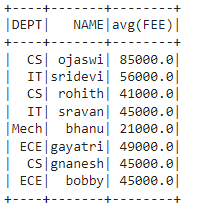We can also  groupBy and aggregate on multiple columns at a time by using the following syntax:

dataframe.groupBy(“group_column”).agg( max(“column_name”),sum(“column_name”),min(“column_name”),mean(“column_name”),count(“column_name”)).show()

We have to import these agg functions from the module sql.functions.

Example:

## Python3

 `# importing module``import` `pyspark`` ` `# import sum, min,avg,count,mean and max functions``from` `pyspark.sql.functions ``import` `sum``, ``max``, ``min``, avg, count, mean`` ` `# importing sparksession from pyspark.sql module``from` `pyspark.sql ``import` `SparkSession`` ` `# creating sparksession and giving an app name``spark ``=` `SparkSession.builder.appName(``'sparkdf'``).getOrCreate()`` ` `# list  of student  data``data ``=` `[[``"1"``, ``"sravan"``, ``"IT"``, ``45000``],``        ``[``"2"``, ``"ojaswi"``, ``"CS"``, ``85000``],``        ``[``"3"``, ``"rohith"``, ``"CS"``, ``41000``],``        ``[``"4"``, ``"sridevi"``, ``"IT"``, ``56000``],``        ``[``"5"``, ``"bobby"``, ``"ECE"``, ``45000``],``        ``[``"6"``, ``"gayatri"``, ``"ECE"``, ``49000``],``        ``[``"7"``, ``"gnanesh"``, ``"CS"``, ``45000``],``        ``[``"8"``, ``"bhanu"``, ``"Mech"``, ``21000``]``        ``]`` ` `# specify column names``columns ``=` `[``'ID'``, ``'NAME'``, ``'DEPT'``, ``'FEE'``]`` ` `# creating a dataframe from the lists of data``dataframe ``=` `spark.createDataFrame(data, columns)`` ` `# Groupby with DEPT  with sum() , min() , max()``dataframe.groupBy(``"DEPT"``).agg(``max``(``"FEE"``), ``sum``(``"FEE"``),``                              ``min``(``"FEE"``), mean(``"FEE"``), ``                              ``count(``"FEE"``)).show()`

Output: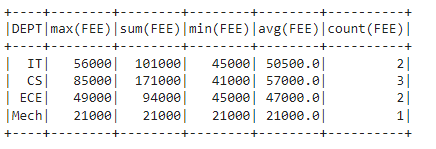My Personal Notes arrow_drop_up